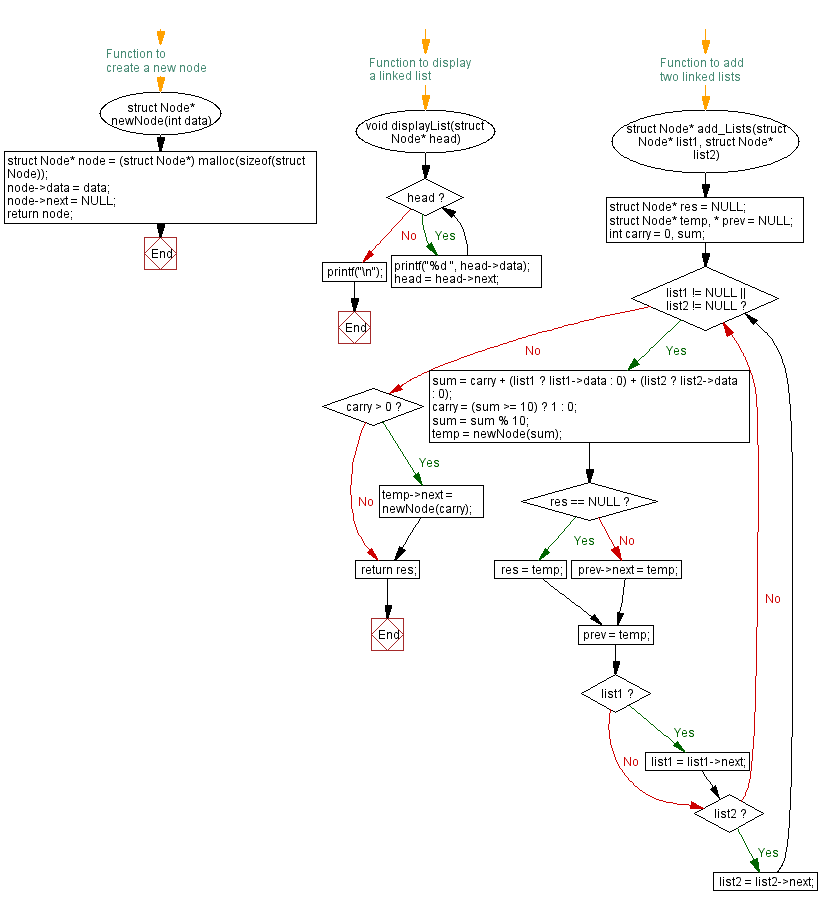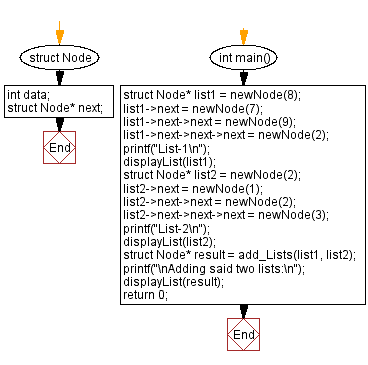## C Singly Linked List : Exercise-22 with Solution

Write a C program that takes two linked lists of numbers. Each node contains a single digit and returns the sum of those numbers of said linked lists as a linked list.

Sample Solution:

C Code:

``````#include<stdio.h>
#include <stdlib.h>

struct Node {
int data;
struct Node* next;
};

// Function to create a new node
struct Node* newNode(int data) {
struct Node* node = (struct Node*) malloc(sizeof(struct Node));
node->data = data;
node->next = NULL;
return node;
}

// Function to display a linked list
}
printf("\n");
}

struct Node* add_Lists(struct Node* list1, struct Node* list2) {
struct Node* res = NULL;
struct Node* temp, * prev = NULL;
int carry = 0, sum;

while (list1 != NULL || list2 != NULL) {
sum = carry + (list1 ? list1->data : 0) + (list2 ? list2->data : 0);

carry = (sum >= 10) ? 1 : 0;
sum = sum % 10;

temp = newNode(sum);

if (res == NULL)
res = temp;
else
prev->next = temp;

prev = temp;

if (list1) list1 = list1->next;
if (list2) list2 = list2->next;
}

if (carry > 0)
temp->next = newNode(carry);

return res;
}

int main() {
struct Node* list1 = newNode(8);
list1->next = newNode(7);
list1->next->next = newNode(9);
list1->next->next->next = newNode(2);
printf("List-1\n");
displayList(list1);
struct Node* list2 = newNode(2);
list2->next = newNode(1);
list2->next->next = newNode(2);
list2->next->next->next = newNode(3);
printf("List-2\n");
displayList(list2);
struct Node* result = add_Lists(list1, list2);
displayList(result);
return 0;
}
```
```

Sample Output:

```List-1
8 7 9 2
List-2
2 1 2 3

0 9 1 6
```

Flowchart :C Programming Code Editor:

What is the difficulty level of this exercise?

﻿